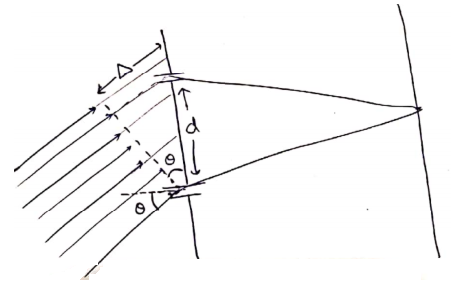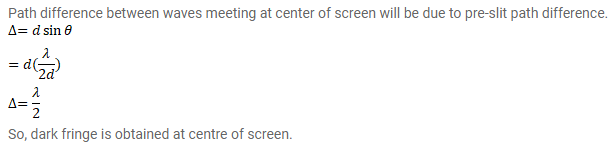# A parallel beam of monochromatic light is used in a Young'sQuestion:

A parallel beam of monochromatic light is used in a Young's double slit experiment. The slits are separated by a distance d and the screen is placed parallel to the plane of the slits. Show that if the incident beam makes an angle $\theta=\sin ^{-1}\left(\frac{\lambda}{2 d}\right)$ with the normal to the plane of the slits, there will be a dark fringe at the centre ${ }^{P_{0}}$ of the pattern.

Solution: